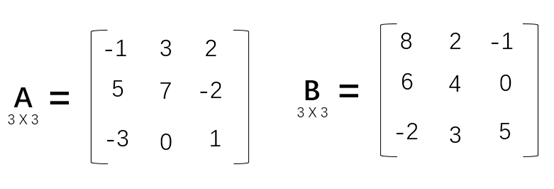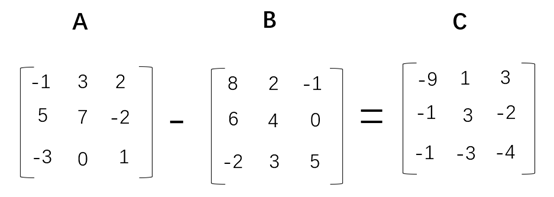1702字，阅读需时6分钟A+B计算过程如下所示：A-B计算过程如下所示：-1-（-7） = -1 + （（-1）（-7））= 6

A-B = A + （-1）* B

（-1）* B的计算过程如下所示：```#定义矩阵A和B
A = [[-1,3,2],[5,7,-2],[-3,0,1]]
B = [[8,2,-1],[6,4,0],[-2,3,5]]

#定义矩阵C，存储A+B的结果
C = [[0,0,0],[0,0,0],[0,0,0]]

#定义矩阵D，存储A-B的结果
D = [[0,0,0],[0,0,0],[0,0,0]]

# 遍历A矩阵的行
for i in range(len(A)):
# 遍历A矩阵的列
for j in range(len(A)):
C[i][j] = A[i][j] + B[i][j]
D[i][j] = A[i][j] - B[i][j]

#输出C矩阵
for r in C:
print(r)

#输出D矩阵
for r in D:
print(r)```

```import numpy as np

#定义矩阵A和B
A = np.array([[-1,3,2],[5,7,-2],[-3,0,1]])
B = np.array([[8,2,-1],[6,4,0],[-2,3,5]])

#计算A+B
print(A+B)

#计算A-B
print(A-B)```

NumPy对矩阵的初始化也是用了两层嵌套列表，计算过程就简单多了，矩阵的加减运算类似两个数的加减运算。